# Coordinate Geometry

Go back to  'Learn'

 1. Introduction 2. What is Coordinate Geometry? 3. Cartesian Plane/Coordinate plane 4. Equation of a line 5. Examples/Activities 6. Summary 7. FAQs

15 September 2020

## Introduction

Geometry is one of the oldest branches in the field of Mathematics. The word ‘Geometry’ is borrowed from Greek, which literally means “Earth Measurement’. Geometry is the study of shapes, sizes, positions, and spatial relationships among different objects.

## What is Coordinate Geometry?

Coordinate or Analytic geometry is a system where an ordered pair of numbers are used to describe the positions of points on a plane, thus helping us to locate points in space.

The (pair of) numbers used to locate a point in a plane are called Coordinates.

## Cartesian Plane/Coordinate plane

The $$2$$- dimensional plane where the coordinates are plotted, in order to define the position of an object is called the Cartesian Plane or Coordinate Plane.

A Cartesian plane has two scales x and y.

X - Axis

X axis runs from left to the right end of the plane (similar to a row in a table)

Y- Axis

Y axis runs from top to bottom and perpendicular to the X axis (similar to a column in a table)

Origin

The point where the X axis and the Y axis meet is called the Origin. The values of x and y at the origin is $$(0,0)$$.

Let us see how a typical Cartesian plane looks like.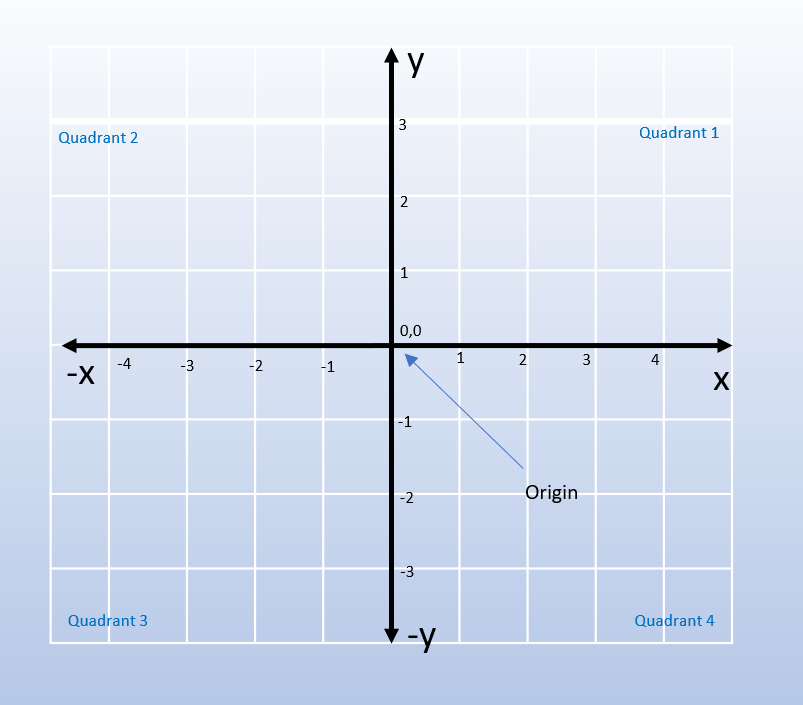In the x-axis, values to the right of the origin point are positive and the values to the left of the origin point are negative.

Similarly, in the y-axis all values above the origin point are positive and all the values below the point are negative.

Any point’s location on the plane is given by a pair of numbers. The first number is the value on the x-axis and the second number is the value on the y-axis.

The x and the y axes divide the plane into four quadrants.

• The part of the plane made by positive x and y axes is called the First Quadrant

• The part of the plane made by negative x-axis and positive y-axis is called the Second Quadrant

• The part of the plane made by negative x and y axes is called the Third Quadrant

• The part of the plane made by positive x-axis and negative y-axis is called the Fourth Quadrant

The quadrants are numbered in an anti-clockwise direction.

Let us see an example of point A in the plane, as shown below.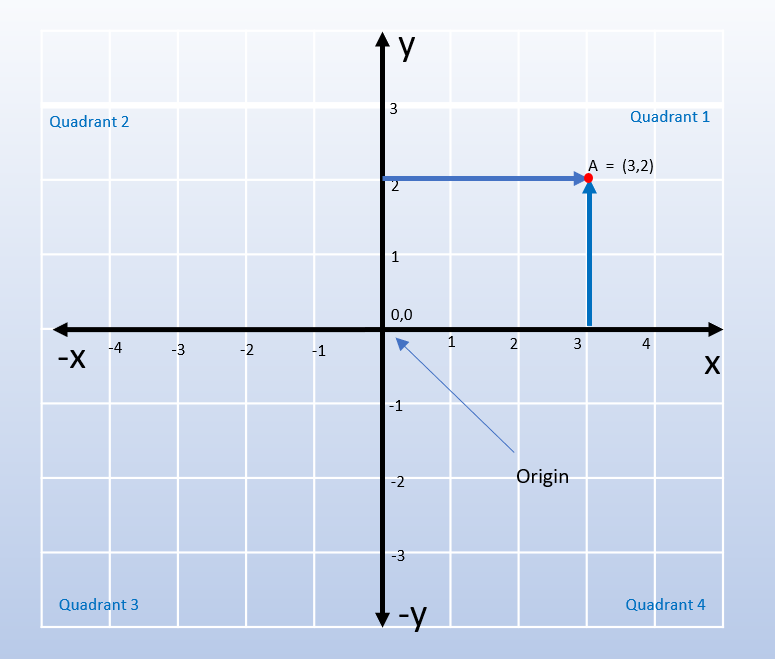To find the coordinate for the point A in the plane, we need to check the corresponding points on the x-axis and the y-axis, which corresponds to $$3$$ and $$2.$$

So, the coordinate for point A is (3,2).## Equation of a line

### What is a line?

A line is a straight one-dimensional figure made by two or more sets of points. It extends on both the sides.

A line can be represented by two or more points on a Cartesian plane. Let us see an example below.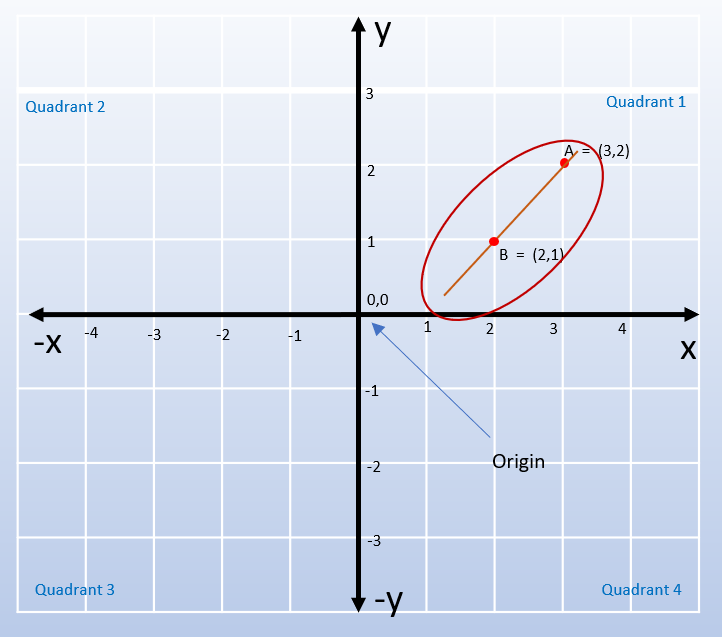In the figure shown above, we have drawn a line passing through two points A and B.

The point A’s coordinate is $$(3,2)$$

The point B’s coordinate is $$(2,1)$$

Only one line can pass through these points. Thus, a line can be uniquely defined by $$2$$ points on a plane.

### A point and a Slope

A line can also be represented by only one point and a slope.

Slope

A slope gives information about the direction/ steepness of the line. It is usually denoted by the letter m. It is also called the gradient of a line.

Slope (m) is calculated using this formula.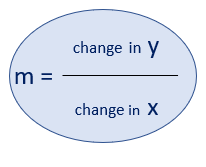Since the slope gives information about the direction of a line, it is sufficient to know only one other coordinate point (instead of $$2$$ coordinate points) to construct a line in a plane.

Positive slope

When y increases with an increase in x, the line rises upwards. Hence it is called a positive slope and the value of m will be a postive number.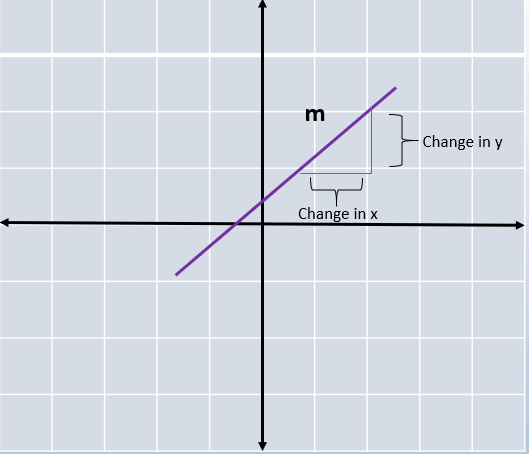Negative slope

When y decreases with an increase in x, the line slopes downwards. Hence it is called a negative slope and the value of m will be a negative number.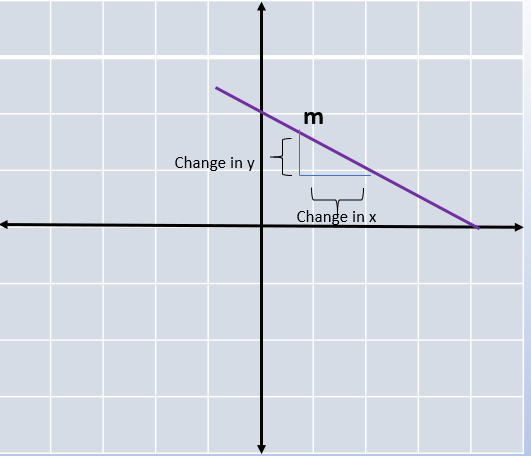Zero slope

When there is no change in the value of y with an increase in x, then it is called a zero slope. The slope m of this horizontal line is zero.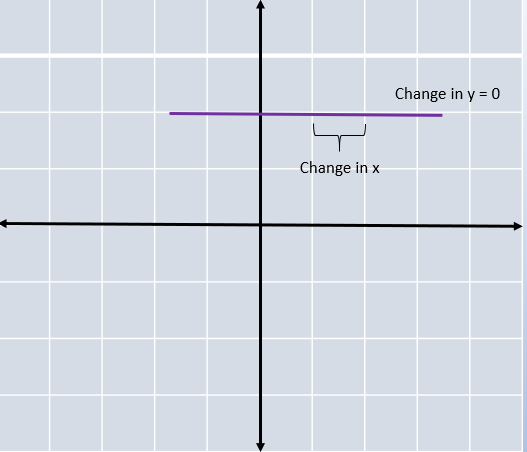Y intercept

The y intercept is a coordinate point (x,y) where x $$= 0.$$ In other words, it is the point where a line touches the y axis.

X intercept

The x intercept is a coordinate point (x,y) where y $$= 0.$$ In other words, it is the point where a line touches the x axis.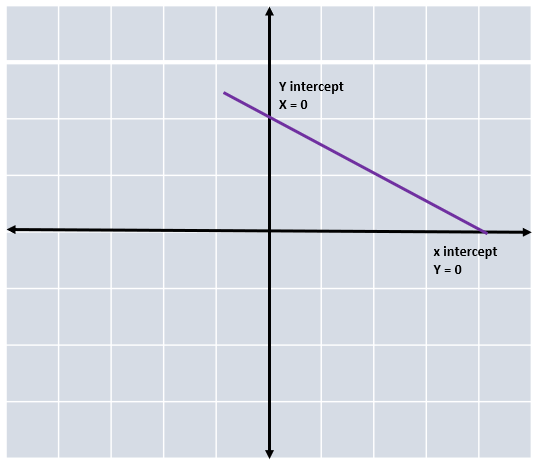### Equation of a straight line (slope – intercept form)

The equation of a straight line in the slope – intercept form is given by the following formula.

 $$\rm{y} = \rm{mx} + \rm{b}$$

Where,

y = y coordinate

x = x coordinate

m = slope of the line

b = y intercept (value of y, when x $$= 0$$ )

### Equation of a straight line (slope – point form)

 $$\rm{y} - \rm{y}1 = m(x - x1)$$

Where,

x1, y1 = known coordinate point

m = slope

x, y = any other point on the line

### Equation of a straight line (general form of a line)

 $$\rm{Ax} + \rm{By} + \rm{c} = 0$$

Where,

A,B, and C are constants

x, y = any other point on the line

## Examples, Activities and Slope intercept Worksheet

### LOCATE THE FOLLOWING POINTS ON A GRAPH

 Coordinates A $$(2,3)$$ B $$(-1, -1)$$ C $$(3, -2)$$ D $$(-3, 3)$$ E $$(0,1)$$ F $$(1, 0)$$ G $$(0, -3)$$ H $$(-4,0)$$ I $$(4, -3)$$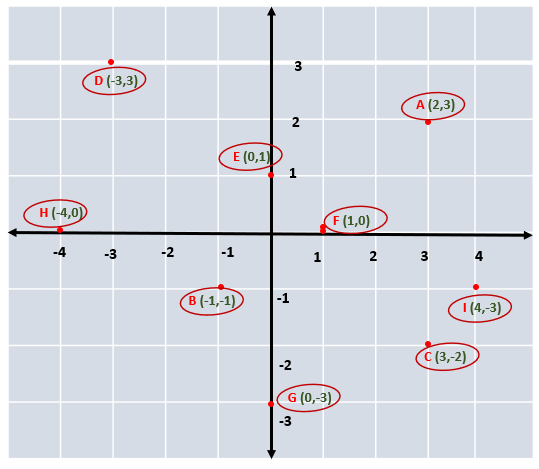### Activity

Plot the following points on a graph sheet.

 Coordinates $$(3,3)$$ $$(0,4)$$ $$(7, 4)$$ $$(2, 0)$$ $$(5,5)$$ Origin point

Are you able to find the x and y intercepts out of these points?

Can you find out which of these points make a square shape when joined with the x and y axes?

### FIND THE SLOPE OF A LINE WITH TWO COORDINATE POINTS A AND B MARKED AS BELOW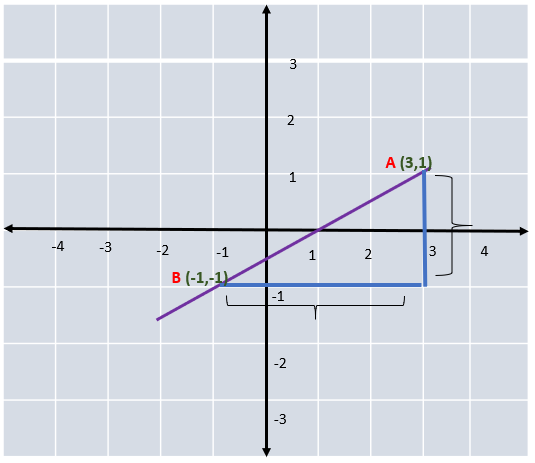m = (change in y) / (change in x)

Changes in the value of x and y are calculated between the two points A and B.

A $$= (3,1)$$

B $$= (-1, -1)$$

Change in y $$= 1 – (-1) = 1 + 1 = 2$$

Change in x $$= 3 – (-1) = 3 + 1 = 4$$

So, slope (m)$$= 2 / 4 = 0.5$$

### Activity

Find the slopes for the following coordinates. Also find if they are positive, negative or zero slopes.

 Coordinates $$(2,3)$$ $$(2,0)$$ $$(0,0)$$ $$(1,5)$$ $$(4,4)$$ $$(7,7)$$ $$(6,3)$$ $$(1,3)$$ $$(10,4)$$ $$(0,4)$$

### Find one or more coordinate points of a line when we have information on y intercept and the slope

Let us take an example with the slope of a line as $$-5$$ and y intercept as $$3.$$

From the equation of a line (slope – intercept form),

y = mx + b

we can form the following equation

y $$= -5x + 3$$

We can find multiple coordinate points for this line by substituting various values for x.

Let us find one such point now.

When x $$= 1,$$

y $$= (-5 x 1) + 3 = -2$$

So, the line passes through the point $$(1, -2)$$

### Activity

Can you try to find more coordinate points for this line and plot them on a graph?

## Summary

The Coordinate system helps in representing geometrical shapes in terms of numbers. It allows the study of algebraic equations on graphs. Coordinate Geometry has multitude of applications in the real-world including Aviation, Space science and Engineering.

We have covered the basic concepts of Coordinate geometry in this article.

Written by Gayathri Sivasubramanian, Cuemath Teacher

## What is the slope of a line?

The slope of a line gives information regarding the direction of the line in terms of how steep the line is. Please refer Slope  for more information.

## What is Abscissa?

Abscissa is the x coordinate of a point in the plane. To put it simply, this is the distance of a point horizontlly from the y – axis.

## What is an Ordinate?

Ordinate is the y coordinate of a point in the plane. This is the distance of a point vertically from the x – axis.

## What is the plural of axis?

The plural of axis is axes.

## What is a Cartesian plane?

The 2- dimensional plane where the coordinates are plotted, in order to define the position of an object is called the Cartesian Plane or Coordinate Plane.

## Where do we use Coordinate geometry in real-life?

• ✓  Used in photo editing and drawing/painting softwares in computers

•   Helps in locating the exact positions of aircrafts

• ✓  Used in map projections. For example , to convert the 3D surface of the earth to a 2D map, coordinate geometry is used to mark every point on the globe

•   Latitudes and Longitudes are marked using the same principle

•   Used extensively while drawing the sketch/plan of a building for the purposes of construction

GIVE YOUR CHILD THE CUEMATH EDGE
Access Personalised Math learning through interactive worksheets, gamified concepts and grade-wise courses